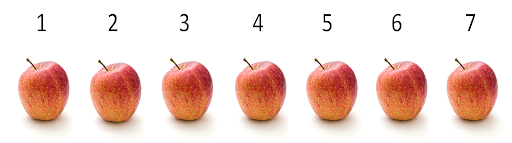Whole Numbers

# 3 Subtracting Whole NumbersAs his company grows, Harpreet asks Jamieson to bid on more jobs. Harpreet has had to hire a few plumbers to help with the work, as things are going well for the business and profits are climbing. It’s going so well that they have had to turn down some of the jobs they are bidding on (and getting).

In fact, last month, they bid on 17 jobs and got all of them. They didn’t figure they would get all 17 and actually had to turn down 5 of the jobs, leaving them with 12 jobs. This is an example of subtracting whole numbers.The key to subtracting whole numbers is to find the difference between the two numbers. If we started with 17 jobs, and Harpreet turned down 5 of those jobs, then the number of jobs he would have taken is 12. We could also look at it as 5 being the difference between the number of jobs he got and the number of jobs he took. We could write this formula down as something like the following:

$\LARGE17-5=12$

OR

$\LARGE17-2=5$

We’ll use the first way it is written down as our example, and then what we’ll do is change that into another format that will be easier to work with.What you might note is that the way the question is written is similar to how we worked the equation when we were adding whole numbers. Writing it this way gives us a better representation of the ones and the tens columns, which we’ll need to use when working through the question.Subtracting, like adding, requires us to work through each of the columns one by one until we reach our final answer. We’ll answer this question visually in order to get the picture.Remove (or subtract) 5 of those apples, and you are left with 2 apples.That takes care of the ones columns. We started with 7 apples, then we subtracted 5 apples, leaving us with 2 apples in the ones column. We would say that 2 is the difference between 7 and 5.Now off to the tens column.What is interesting to note here is that there is only one number in the tens column, and that happens to be the number 1. This makes things easy, as there is no work for us to do. We just move the 1 down into the tens column of the answer, and we then have our final answer.Okay, that was pretty straightforward. Now we’ll try something a little more challenging.

Example

Imagine things worked out differently for Harpreet and Jamieson. Let’s say that, of those 17 jobs they bid on and successfully got, they had to turn down 9 of them. How many of those jobs would they have taken? Before you continue reading and see the answer, try and visualize what it would look like when we put those numbers into the formula. Do you see the problem?If we were to start with the ones column as we did in the last example, the problem would show up right away. The problem is that, if you try to subtract 9 from 7, you would end up below zero. So we have to come up with some method of subtracting that compensates for that.

$\LARGE7-9=\text{less than zero}$What we end up doing is borrowing from the tens column. We would end up with something that looked like this:When we borrow from the tens column, we are borrowing a value of ten and adding it to the ones column to help the ones. We end up with 17 in the ones column, which is now more than enough to deal with the 9 being subtracted. Also, whatever value we had in the tens column (in this case, it was a 1) is reduced by 1 to account for the fact that it has been borrowed.

Example

We’ll go through another example in which we return to our plumbers, Harpreet and Jamieson. The three employees they hired are Dixon, Kavanir, and Arman, and it’s a good thing they hired them. Those 17 jobs they originally bid on involved the installation of 246 fixtures, including bathtubs, toilets, and sinks. Harpreet and Jamieson could not do all that work by themselves.

But, due to the fact that they turned down 5 jobs, they won’t need to install 75 of those fixtures, so with the help of the three new employees, they should be able to complete all the jobs. The question is, “How many fixtures will they have to install?”As usual, start with the mathematical formula that allows us to properly answer the question. But this time, we’ll go through the process using steps, so that when you look back, you can see how it breaks down.

You may have noticed in the first couple of sections that we often go through examples using steps. This is done to break down a large question into manageable parts. If you follow this idea when working through math problems, it can help keep you on track.

Okay, back to the problem:

Step 1: Put the question into a format that is easy to work with.Step 2: Subtract the ones. In this case, we have 6 minus 5, which equals 1.Step 3: Subtract the tens. Here, we have the issue wherein the number on top (4) is less than the number on the bottom (7), so we have to borrow from the hundreds. The 2 in the hundreds column has to be reduced by 1, and then that 1 is added to the tens. We end up with 14 minus 7, which equals 7.Step 4: Subtract the hundreds. In this case, we only have the 1, so 1 minus 0 is 1.# Practice Questions

Try a couple example questions yourself and check the video answers to see how you did.

Question 1

Question 2

##